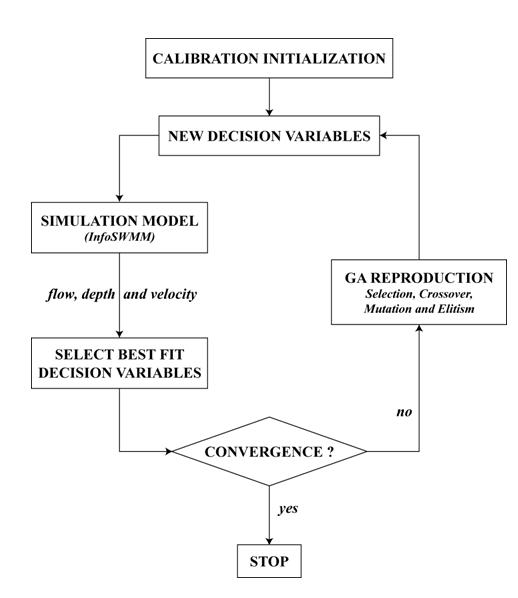#INFOSWMM

# Calibration Methodology in InfoSWMM and InfoSWMM SA

Calibration Methodology

The successful application of a sewer collection system model to the planning, design, and management of urban stormwater systems is highly dependent upon how well the model is calibrated and how well the model mimics the reality. Model calibration consists of fine tuning of model parameters until the model simulates field conditions to an established degree of accuracy. Fine-tuning of the model entails making adjustments to the model parameters to obtain the desired output data. The degree of accuracy refers to the difference between simulated and actual values and is used to establish a level of confidence in the model. It is normally expressed as a percent difference (typically [ 10 percent) between model predictions and actual measurements. Calibration is important to establish model credibility, create a benchmark, produce a predictive tool, increase knowledge and understanding of the system and its operations, and to discover errors or unknown conditions in the field.

Periodic re-calibration of models is also necessary not only when major new facilities are added to the system or when system operations change, but also to learn more about the system so that informed decisions can be effectively made to improve system operations and performance.

InfoSWMM Calibrator provides a fully automated approach to accurate and efficient stormwater management model calibration. The program makes use of a variation of the genetic algorithm optimization technology enriched with global search control strategies and closely coupled with InfoSWMM for maximum efficiency, performance and reliability.

1 CALIBRATION FORMULATION

When calibrating a stormwater management model, the goal is to determine the best set of InfoSWMM model parameters which produces the lowest overall deviation between the numerically simulated model results and the observed field data at user specified locations in the system. Observed field data can include link flow, link depth, and link velocity measurements. These measurements could be taken at one or more links in the system.

Grouping is very useful in the calibration process. InfoSWMM calibrator has five group types: subcatchment group, soil group, aquifer group, RDII group, and conduit group. Grouping has to be made accounting for similarity in the characteristics of the elements. For example, if the modeler is interested in calibrating subcatchment width s/he may need to group subcatchments depending on their similarity in shape/size so that subcatchments in the group may use the same multiplying factor during the calibration. To calibrate Manning’s roughness parameter for conduits, one may need to group conduits together depending on similarity in their characteristics such as material, date of installation, location, and diameter. It is assumed that all elements within a group will have an identical multiplying factor for the parameter being calibrated. The multiplying factor would be multiplied by the original parameter value assigned to each of the individual elements to determine actual value of parameter to be used during the calibration process.

InfoSWMM Calibrator casts the calibration problem as an implicit nonlinear optimization problem, subject to explicit inequality and equality constraints. It computes optimal model parameters within user-specified bounds, such that the deviation between the model predictions and field measurements is minimized. The optimal model calibration problem is thus governed by an objective function and its associated set of constraints.

1.1 OBJECTIVE FUNCTIONS

The objective of the optimal calibration problem is to minimize the numerical discrepancy between the observed and predicted values of link flow, link depth, and/or link velocity at various locations in the system. Any of the following nine different mathematical objective functions can be used in InfoSWMM Calibrator.

Root Mean Square Error (RMSE)In the ideal condition when corresponding observed and simulated values exactly match, value of RMSE will be zero.

Simple Least Square Error (SLSE)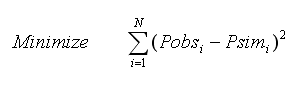This performance evaluation function could assume values ranging from zero (best fit) to ∞ (poor fit), and it tends to favor large errors and large (i.e., peak) flows.

Mean Simple Least Square Error (MSLSE)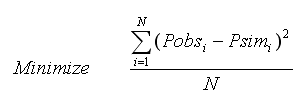This performance evaluation function could assume values ranging from zero (best fit) to ∞ (poor fit), and it tends to favor large errors and large (i.e., peak) flows.

Difference in Total Volume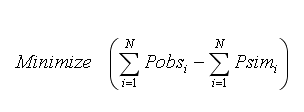Difference in total volume could range from -∞ (poor performance) to ∞ (poor performance), the ideal value being zero (i.e., exact match between total simulated volume and total observed volume).

Nash-Sutcliffe Efficiency Criterion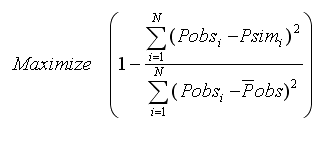This efficiency criterion could assume values from -∞ (poor performance) to 1 (perfect model).

R-Square (R2)R2 value varies from zero (indicates worst fit) to unity (indicates perfect fit).

Modified Coefficient of Efficiency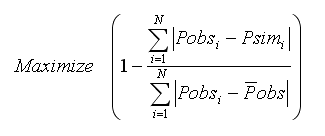The modified coefficient of efficiency could assume values from -∞ (poor performance) to 1 (perfect model).

Dimensionless Root Mean Square Error (DRMSE)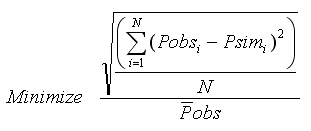In the ideal condition when corresponding observed and simulated values exactly match, value of DRMSE will be zero.

Dimensionless Simple Least Square Error (DSLSE)where N designates the total number of measurements available, Pobsi represents the observed measurement values at time i; Psimi is the model simulated values at time i; is mean of the measured values; is mean of the simulated values.

This performance evaluation function could assume values ranging from zero (best fit) to ∞ (poor fit), and it tends to be independent of length of records and it favor large errors and large (i.e., peak) flows.

It is believed that complete assessment of model performance should include at least one relative error measure (e.g., modified coefficient of efficiency) and at least one absolute error measure (e.g., root mean square error) with additional supporting information (e.g., a comparison between the observed and simulated mean and standard deviation).

The number of field measurements defining the objective function must be greater than or equal to the number of calibration variables. It is expected that the accuracy of the model calibration would be increased by the use of a large number of field measurements. The decision variables could be any one or more of about fifty InfoSWMM parameters. These decision variables are automatically adjusted to minimize the objective function selected while satisfying a set of implicit and explicit constraints.

1.2 IMPLICIT SYSTEM CONSTRAINTS

The implicit constraints on the system correspond to equations that govern/simulate the underlying hydrologic, hydraulic and water quality processes including conservation of mass and conservation of momentum at various scales such as node, and/or link, and/or the entire system. Each function call to InfoSWMM with a set of decision variables returns the simulated values for link flow, link depth, and link velocity that will be compared with corresponding measured data.

1.3 EXPLICIT CONSTRAINTS

The explicit bound constraints are used to set minimum (lower) and maximum (upper) limits on the calibrable InfoSWMM parameters. For example, for each conduit group G (where a single conduit may constitute a group) Manning’s roughness coefficient for a conduit in the group may be bound by an explicit inequality constraint as follows:where kmin designates the lower bound multiplier (minimum value of a multiplier) for roughness coefficient of conduits in conduit group G; kmax represents the upper bound multiplier (maximum value of a multiplier) for roughness coefficient of conduits in conduit group G; and n is roughness coefficient value for conduit i in group G. Please note that each of the conduits in group G could take different values of n. The only value that is same for all conduits in the group is the multiplier k.

It should be noted that the choice of grouping may greatly affect the calculated parameter values as well as the convergence accuracy. It is also expected that the final calculated parameters, will be close to the actual values (i.e., optimal/near optimal values) although they may not be “exact” or absolute optima.

2 GENETIC ALGORITHMS

Genetic algorithms (GA) are an adaptation procedure based on the mechanics of natural genetics and natural selection1. They are designed to perform search procedures of an artificial system by emulating the evolution process (Darwin’s evolution principle) observed in nature and biological organisms. The evolution process is based on the preferential survival and reproduction of the fittest member of a population with direct inheritance of genetic information from parents to offspring and the occasional mutation of genes. The principal advantages of genetic algorithms are their ability to converge expeditiously on an optimal or near-optimal solution without having to analyze all possible solutions available and without requiring derivatives or other auxiliary knowledge.

2.1 OVERVIEW OF GENETIC ALGORITHMS

Genetic algorithms are fundamentally different from traditional optimization methods in terms of the search process. While traditional routines track only a single pathway to the optimal solution, genetic algorithms search from an entire population of possible solutions (individuals). In addition, genetic algorithms use randomized and localized operators as opposed to deterministic rules. Each individual in the population is represented by either a string or a set of real numbers encoding one possible solution. The performance of each individual in the population is measured by its fitness (goodness), which quantifies the degree of optimality of the solution. Based on their fitness values, individuals are selected for reproduction of the next generation. Each new generation maintains its original size. The selected individuals reproduce their offspring by mimicking gene operations of crossover and mutation. After a number of generations, the population is expected to evolve artificially, and the optimal or near optimal solution is ultimately reached.

2.2 COMPONENTS OF GENETIC ALGORITHMS

Standard genetic algorithms involve three basic functions: selection, crossover, and mutation. Each function is briefly described below.

Selection – Individuals in a population are selected for reproduction according to their fitness values. In biology, fitness is the number of offspring that survive to reproduction. Given a population consisting of individuals identified by their chromosomes, selecting two chromosomes to represent parents to reproduce offspring is guided by a probability rule that the higher the fitness an individual has, the more likely the individual will survive. There are many selection methods available including weighted roulette wheel, sorting schemes, proportionate reproduction, and tournament selection.

Crossover - Selected parents reproduce the offspring by performing a crossover operation on the chromosomes (cut and splice pieces of one parent to those of another). In nature, crossover implies two parents exchange parts of their corresponding chromosomes. In genetic algorithms, a crossover operation makes two strings swap their partial strings. Since more fit individuals have a higher probability of producing offspring than less fit ones, the new population will possess, on average, an improved fitness level. The basic crossover is a one-point crossover. Two selected strings create two offspring strings by swapping the partial strings, which is cut by one randomly sampled breakpoint along the chromosome. The one-point crossover can easily be extended to k-point crossover. It randomly samples k breakpoints on chromosomes and then exchanges every second corresponding segments of two parent strings.

Mutation - Mutation is an insurance policy against lost genes. It works on the level of string genes by randomly altering gene value. With small probability, it randomly selects one gene on a chromosome then replaces the gene by a randomly selected value. The operation is designed to prevent GA from premature termination namely converging to a solution too early.

Elitism - The selection and crossover operators will tend to ensure that the best genetic material and the components of the fittest chromosomes will be carried forward to the next generation. However, the probabilistic nature of these operators implies that this will not always be the case. An elitist strategy is therefore required to ensure that the best genetic material will not be lost by chance. This is accomplished by always carrying forward the best chromosome from one generation to the next.

The flowchart below illustrates the optimal calibration process.